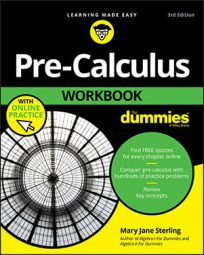##### Pre-Calculus Workbook For DummiesWhether an exponential equation contains a variable on one or both sides, the type of equation you’re asked to solve determines the steps you take to solve it.

The basic type of exponential equation has a variable on only one side and can be written with the same base for each side. For example, if you’re asked to solve 4x – 2 = 64, you follow these steps:

1. Rewrite both sides of the equation so that the bases match.

You know that 64 = 43, so you can say 4x – 2 = 43.

2. Drop the base on both sides and just look at the exponents.

When the bases are equal, the exponents have to be equal. This step gives you the equation x – 2 = 3.

3. Solve the equation.

This example has the solution x = 5.

If you must solve an equation with variables on both sides, you have to do a little more work (sorry!). For example, to solve 2x – 5 = 8x – 3, follow these steps:
1. Rewrite all exponential equations so that they have the same base.

This step gives you 2x – 5 = (23)x – 3.

2. Use the properties of exponents to simplify.

A power to a power signifies that you multiply the exponents. Distributing the exponent inside the parentheses, you get 3(x – 3) = 3x – 9, so you have 2x – 5 = 23x – 9.

3. Drop the base on both sides.

The result is x – 5 = 3x – 9.

4. Solve the equation.

Subtract x from both sides to get –5 = 2x – 9. Add 9 to each side to get 4 = 2x. Lastly, divide both sides by 2 to get 2 = x.